Iteration Explicit Sequences of Second-Order Equations

We write a function that takes two values (the current, and its antecedent) and uses a discrete step Δ to push the solution into the future. This method uses the current value to estimate the right-hand-side; so it is an Explicit method.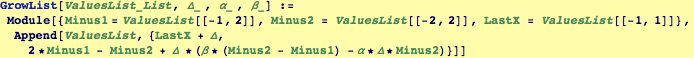Notice that this forward differnce needs TWO consectutive past values before it can calculate the current value, create a function to Appends the current value to a list by using the last two values:

The fact that TWO consecutive values are needed is not surprising. Because this iteration procedure is giving an approximation to the solution of a second-order ODE, we need to make a numerical approximation to a second derivative. THREE points—the two consecutive ones and the current one—are needed to do this. (If only two points were used, you could only compute the slope of the straight line connecting the points; if three points are used, a circle can be fit to the three points and the inverse of its radius gives the curvature.)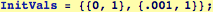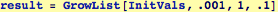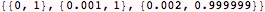Generate a sequence of length 20 from initial values {1,1} for Δ=0.001, α=1, β=0.1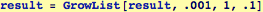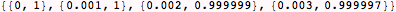Using NestWhile, we can grow the list until the accumulation of the discrete steps reaches a particular value.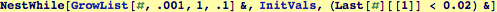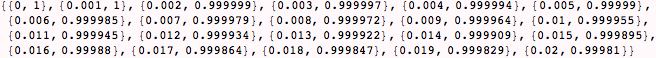Created by Wolfram Mathematica 6.0  (16 November 2007)